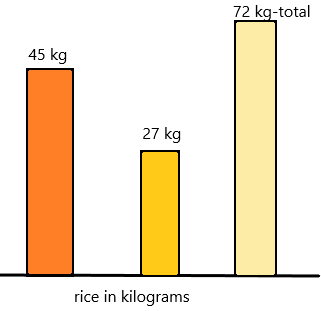# Math in Focus Grade 2 Chapter 8 Practice 5 Answer Key Real-World Problems: Mass

Practice the problems of Math in Focus Grade 2 Workbook Answer Key Chapter 8 Practice 5 Real-World Problems: Mass to score better marks in the exam.

## Math in Focus Grade 2 Chapter 8 Practice 5 Answer Key Real-World Problems: Mass

Solve.

Question 1.
Angelina has two dogs. The masses of the two dogs are 35 kilograms and 67 kilograms. What is the total mass of the two dogs? The total mass of the two dogs is ___ kilograms.
Explanation:
The mass of one dog=35 kg
The mass of another dog=67 kg
The total mass of two dogs=X
X=35+67
X=102.
Therefore, the total mass of the two dogs is 102 kilograms.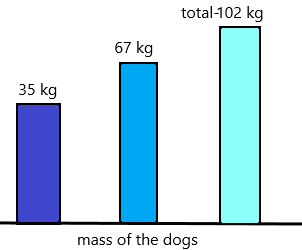Question 2.
Miguel has a mass of 32 kilograms. He is 5 kilograms lighter than Sal. What is Sal’s mass?
Sal’s mass is ___ kilograms.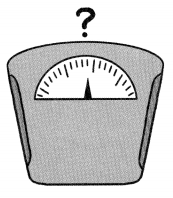Explanation:
The mass of Miguel has=32 kg
The number of kilograms of Miguel lighter than sal=5
The sal’s mass=X
Here Miguel’s mass is 5 kilograms less than sal. Now we have to calculate the sal’s mass.
Add 5 to Miguel’s mass then we get the sal’s original mass.
X=32+5
X=37.
Therefore, the mass of sal’s has 37 kg.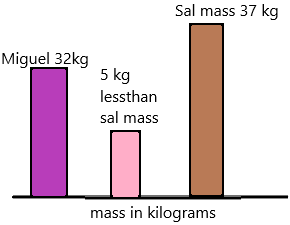Question 3.
Mr. Souza needs 400 grams of clay to make a small statue. He has only 143 grams of clay. How much more clay does he need? He needs ___ grams more clay.
Explanation:
The number of grams Souza needs clay to make a statue=400
The number of grams she has now=143.
The number of more clay she needs=X
subtract total clay she needs and she has clay now
X=400-143
X=257.
Therefore, 257 grams more is needed.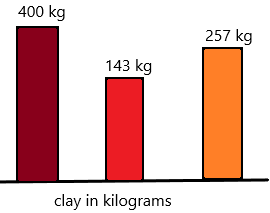Solve.

Question 4.
Ali has a mass of 25 kilograms. Tyrone is 6 kilograms heavier than Ali. What is their total mass?
Their total mass is ___ kilograms.
Explanation:
The mass of an Ali =25 kg
The mass of Tyrone=25+6=31.   ( 6kgs heavier than Ali so I added 6 to 25).
The total mass of both=25+31=56.
Therefore, Their total mass is 56 kilograms.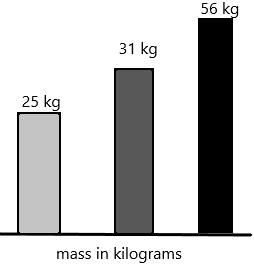Question 5.
Twyla buys a bag of onions with a mass of 750 grams. She uses 100 grams of the onions for lunch. She uses 480 grams of onions for dinner. What is the mass of the onions that are left?
The mass of onions left is ___ grams.
Explanation:
The mass of onions Twyla buys=750
The number of grams of onions she uses for lunch=100
The number of grams of onions she uses for dinner=480
The total number of grams of onions she used overall=100+480=580
The mass of onions she left with her=X
Subtract the mass of onions she buys and the mass of used onions.
X =750-480
X=170
Therefore, 170 grams of onions she had left with her.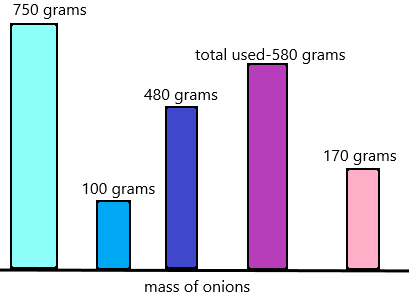Question 6.
Tim sells 45 kilograms of rice on Monday. He sells 18 kilograms less rice on Tuesday than on Monday. How much rice does he sell in all on the two days?
He sells ___ kilograms of rice in all on the two days.